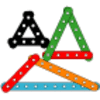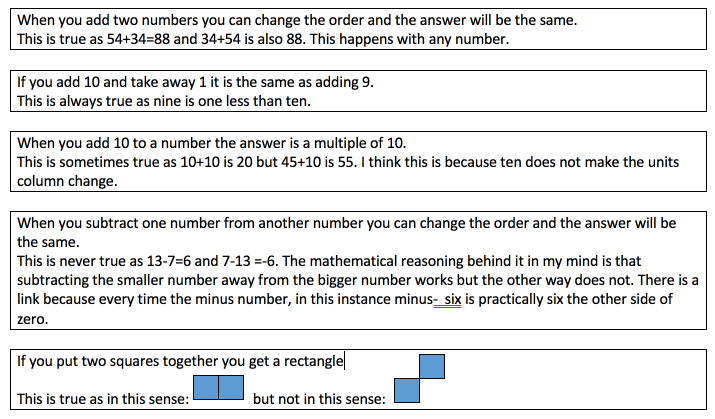#### You may also like### I'm Eight

Find a great variety of ways of asking questions which make 8.### Let's Investigate Triangles

Vincent and Tara are making triangles with the class construction set. They have a pile of strips of different lengths. How many different triangles can they make?### Noah

Noah saw 12 legs walk by into the Ark. How many creatures did he see?

# Always, Sometimes or Never? KS1

##### Age 5 to 7 Challenge Level:
E.'B'.A. from B J S in England sent in the following solution.

When you add two numbers, you can change the way round they are and the answer will always be the same. This is true for it will always give the same answer as you're adding it on.

If you add ten to a number and then take away 1 it is the same as adding 9. This is always true because we can never change the fact that 10-1 is 9.

When you add ten to a number it will always be a multiple of ten. The answer to this is sometimes because if I add ten to 25 it will give me 35 which is not a multiple of ten. It will only work with certain numbers.

When you subtract one number from another number and change the order the answer will be the same. This answer is also a sometimes because 9 - 7 is  2, 7 - 9 is a -2. This will only work if the numbers are the same.

SHAPES

If you put two squares together you get a rectangle. This is always true because no matter which way you put it, the width is always going to be double the height.

All 3-D shapes have more than four faces. This is never right because a cylinder as three faces - two flat and a curved.

When you cut a square in half you get a triangle. The answer to this question is sometimes as it depends which way you dissect it. If it is diagonally, then yes you will get a triangle.

Four sided shapes are called squares. This is never right except from a square. A four side shape is called a quadrilateral.

All three sided shapes are called triangles. This is right as all three sided shapes have to be triangles. They might be irregular but they are all triangles.

Scout from James Allen's Prep School sent in the following:Thank you both for your excellent solutions.